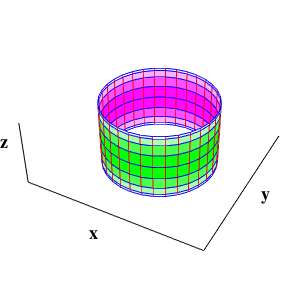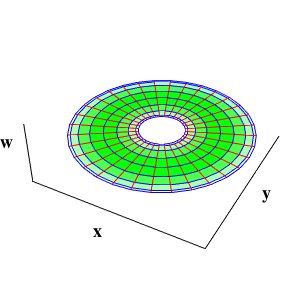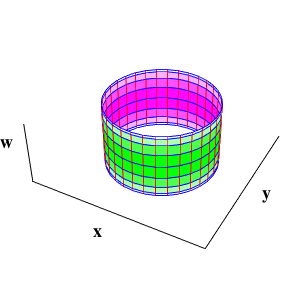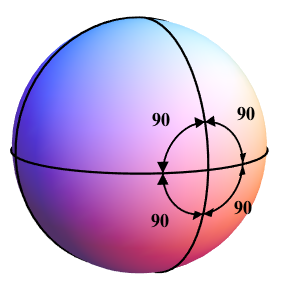# Diaspora

## Chapter 2: Truth Mining

### (Detailed version)

The standard way to embed a torus in 3 dimensions is:

 (x, y, z) = ((a – b cos B) cos A, (a – b cos B) sin A, b sin B) (1)

where a and b are the major and minor radii of the torus, and A and B are angles that vary from 0 to 2 π radians. This is shown below left.Torus (1) in (x, y, z) coords Torus (2) in (x, y, z) coords, w shown as shading

But the torus can also be embedded in 4 dimensions, as:

 (x, y, z, w) = (a cos A, a sin A, b sin B, b cos B) (2)

This new embedding can be produced by rotating each of the original red meridians 90 degrees into the 4th spatial dimension, converting the variation in distance from the central axis to variation in the w coordinate.

Seen in (x, y, z) coordinates, the meridians start out clearly being circles (above left) and appear to be squashed into vertical lines (above right), but the w coordinate (shown as shading) gives them room to retain their shape.

Seen in (x, y, w) coordinates (with z shown as shading), the meridians start out horizontal (below left) and become vertical in the w direction (below right); in both cases the circles appear as lines, because their varying z coordinate is shown only as shading.Torus (1) in (x, y, w) coords, z shown as shading Torus (2) in (x, y, w) coords, z shown as shading

### (Detailed version)

Any surface can be characterised by its Euler number: if the surface is divided into polygons (or “faces”), the Euler number χ is given by:

 χ = F – E + V

where F is the number of faces, E is the number of edges, and V is the number of vertices. For a sphere divided into 8 triangles:

 F = 8 E = 12 V = 6 χ = 8 – 12 + 6 = 2

The Euler number remains the same even if F, E or V are changed. For example, merging two triangles with a common edge to form a square reduces F by 1, but also reduces E by 1, so χ is unchanged.

To see why the Euler number of any flat surface (with no boundary) that can be divided into a finite number of faces must be zero, consider the special case where all the faces are triangles, sharing complete edges with each other. Then every edge is shared by exactly two triangles, so:

 E = 3 F / 2

If the surface is flat, the sum of the internal angles of all the triangles, T, must total 180 degrees times the number of triangular faces:

 T = 180 F

but this sum must also be reached by adding up the 360-degree angles around every vertex:

 T = 360 V

Equating the two gives:

 V = F / 2 χ = F – E + V = F – (3 F / 2) + (F / 2) = 0

Since a sphere has a Euler number of 2, it can never be made flat.A sphere divided into 8 triangles, with 360 degrees around each vertex The same sphere deformed so that each triangle is Euclidean, with angles summing to 180 degrees

### Euler characteristic applet

The applet below lets you play around with different divisions of the sphere into polygons, to see how the number of faces, edges and vertices change when these features are subdivided or deleted. The initial division comes from a choice of polyhedron, but you can then make as many modifications as you like.

 Truncated Icosahedron Octahedron Cube Dodecahedron Icosahedron

Drag to rotate sphere, click to select features to divide or delete.

 FacesF EdgesE VerticesV Euler characteristicχ = F – E + V Sum of anglesaround all vertices Sum of internalangles of facesif they were flat 32 90 60 2 21 600° 20 880°Diaspora / Chapter 2: Truth Mining (detailed) / created Saturday, 25 October 1997 / revised Friday, 9 August 2019